# Perturbation Theory

Suppose energy is the sum of two terms:

U=U0+U1

and we know how to calculate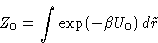How can we calculate partition function Z?

Idea: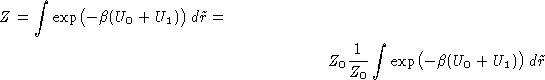But this is exactly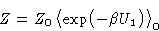(25)
with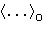--averaging over reference system with energy U0: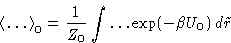For the free energy: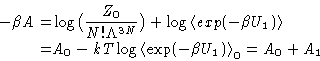(26)
A1 can be expanded in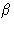, by expanding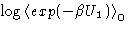.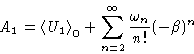(27)
This makes a series in powers of the perturbation energy U1, averaged over the configurations of the unperturbed system. (They are contained in the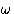's).

Van der Waals Equation of state:
Let's consider the hard-sphere system as a reference, and the attractive part of the molecular interaction v(r) as a perturbation.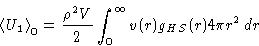Assuming at low densities: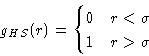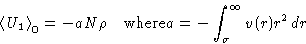For the hard spheres system: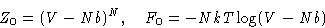Substituting this into (26,27), and differentiating with respect to volume, obtain the Van der Waals equation of state: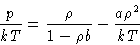Next: Conclusions Up: Liquids. Density Correlation Functions Previous: Distribution Functions

© 1997 Boris Veytsman and Michael Kotelyanskii
Thu Sep 25 23:59:09 EDT 1997